## Indicators

•Cited by SciELO
•Access statistics

## Related links

•Similars in SciELO

## Print version ISSN 1405-7743

### Ing. invest. y tecnol. vol.16 n.3 México Jul./Sep. 2015

Numerical Modeling of the Thomson Ring in Stationary Levitation Using FEM-Electrical Network and Newton-Raphson

Modelación numérica del anillo de Thomson en levitación estacionaria usando circuitos eléctricos, MEF y Newton-Raphson

Guzmán Juan1, González-Montañez Felipe de Jesús2, Escarela-Pérez Rafael3, Olivares-Galván Juan Carlos4, Jiménez-Mondragon Victor Manuel 5

1 Departamento de Energía, Universidad Autónoma Metropolitana (UAM), Unidad Azcapotzalco. E-mail: maestro_juan_rafael@hotmail.com.

2 Departamento de Energía, Universidad Autónoma Metropolitana (UAM), Unidad Azcapotzalco. E-mail: fjgm@correo.azc.uam.mx.

3 Departamento de Energía, Universidad Autónoma Metropolitana (UAM), Unidad Azcapotzalco. E-mail: r.escarela@ieee.org.

4 Departamento de Energía, Universidad Autónoma Metropolitana (UAM), Unidad Azcapotzalco. E-mails: jolivarej_1999@yahoo.com.

5 Departamento de Energía, Universidad Autónoma Metropolitana (UAM), Unidad Azcapotzalco. E-mail: vmjm1986@gmail.com.

Information on the article: received: March 2014,
Reevaluated: June 2014,
Accepted: July 2014.

Abstract

There are a lot of applications of the Thomson ring: levitation of superconductor materials, power interrupters (used as actuator) and elimination of electric arcs. Therefore, it is important the numerical modeling of Thomson ring. The aim of this work is to model the stationary levitation of the Thomson ring. This Thomson ring consists of a copper coil with ferromagnetic core and an aluminum ring threaded in the core. The coil is fed by a cosine voltage to ensure that the aluminum ring is in a stationary levitated position. In this situation, the state of the electromagnetic field is stable and can be used the phasor equations of the electromagnetic field. These equations are discretized using the Galerkin method in the Lagrange base space (finite element method, FEM). These equations are solved using the COMSOL software. A methodology is also described (which uses the Newton-Raphson method) that obtains the separation between coil and aluminum ring. The numerical solutions of this separation are compared with experimental data. The conclusion is that the magnetic coupling of the aluminum ring on the coil can be neglected if the source voltage is high.

Keywords: Thomson ring, levitation, stationary, modeling, FEM.

Resumen

Existen una gran cantidad de aplicaciones del anillo de Thomson: levitación de materiales superconductores, interruptores de potencia (usados como actuadores) y eliminación de arcos eléctricos. Por lo tanto, es importante la modelación del anillo de Thomson. El objetivo de este trabajo es modelar la levitación estacionaria del anillo de Thomson. Este anillo de Thomson consiste de una bobina de cobre con núcleo ferromagnético y un anillo de aluminio enhebrado en el núcleo. La bobina se alimenta por un voltaje cosenoidal para asegura el anillo de aluminio en una posición de levitación estacionaria. En esta situación, el campo electromagnético se puede considerar estable y se pueden emplear las ecuaciones fasoriales del campo electromagnético. Estas ecuaciones se discretizan usando el método de Galerkin en el espacio base de Lagrange (método de elementos finitos, FEM). Estas ecuaciones discretizadas se resuelven usando el código COMSOL. Además, se describe una metodología con la cual se puede obtener la separación entre la bobina y el anillo de aluminio. Esta metodología usa el método de Newton-Rapson. Las soluciones numéricas de esta separación se comparan con datos experimentales. Se concluye que el acoplamiento magnético entre el anillo de aluminio sobre la bobina se puede despreciar si el voltaje de alimentación es alto.

Descriptores: anillo de Thomson, levitación, estacionaria, modelación, MEF.

Introduction

In the electric industry is important to have electric systems of immediate breaking and safe. Some of these electric systems consist of power switchers, which use the Thomson ring (Alferov et al., 2008; Meyer and Rufer, 2006). Other systems utilize the Thomson ring as actuator to eliminate the electric arcs (Li et al., 2010). Other applications of the Thomson ring consist in the levitation of superconductor materials (Patitsas, 2011). Therefore, it is important the numerical modeling of the Thomson ring. The Thomson ring consists of a coil with ferromagnetic core on which an aluminum ring levitates. The coil is fed by a cosine voltage.

The modeling of the electromagnetic field of any electric device (as the Thomson ring) requires of the knowledge of the current density. However, this knowledge cannot be known a priori. It is known a priori the power source voltage instead of current density. In the literature, several methods (Belforte et al., 1985; Bissal et al., 2010; Konrad, 1982; Lombard and Meunier, 1992, 1993; Meunier et al., 1988; Piriou and Razek, 1989) have been developed to calculate the electromagnetic field if the power source voltage is supplied: integro-differential method (Konrad, 1982); direct methods (Belforte et al., 1985; Meunier et ah, 1988; Piriou and Razek, 1989); and methods that use electric networks equations (Barry and Casey, 1999; Bissal et al, 2010; Lombard and Meunier, 1992, 1993). In this work is supposed that the power source voltage is known and the current density is calculated using electric networks equations.

Several studies have analyzed the mathematical and physics models of the Thomson ring. In the work of Bissal et al. (2010) is modeled the dynamic behavior of the Thomson ring, which consist of a coil without ferromagnetic core. In this work, the coil is fed by a capacitor. Barry and Casey (1999) obtained analytical solutions of the force acting on the aluminum ring in a stationary levitated position. In the work of Li et al. (2010) is analyzed the dynamic characteristics of the Thomson ring used as actuator to eliminate the electric arcs. In the work of Patitsas (2011) is developed a new modality of Thomson ring. This modality consisted in keeping the stable levitation of a superconductor sphere immerse in a magnetic field supplied by a coil.

The aim of this work is to analyze the Thomson ring when the aluminum ring is a stationary levitated position. This situation is reached if the coil is fed by a cosine voltage. In the stationary levitation, the state of the electromagnetic field is stable and can be used the phasor equations of the electromagnetic field. These equations are discretized using the Galerkin method. These discretized equations are solved using the COMSOL software (COMSOL, 2008). It is described the methodology (which uses the Newton-Raphson method) that obtains the separation between the coil and the aluminum ring in stationary levitation (mechanical equilibrium). Also, the separation obtained with this methodology is compared with the experimental data for different values of the power source voltage. It is concluded that the magnetic coupling of the aluminum ring on the coil can be neglected if the source voltage is high.

Experimental setup

The Thomson ring used in this work consists of a coil with ferromagnetic core; where an aluminum ring is threaded on the core, as shown in Figure 1. The ferromagnetic core consists in a solid cylinder that is collocated vertically, as is illustrated in Figure 1. In this figure, Zs represents the distance between the coil and the aluminum ring.The coil is made of copper wire and consists of 1140 turns (see, Table 1). This coil is fed by a cosine voltage given by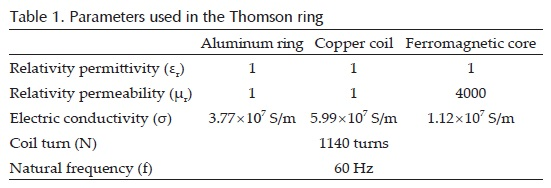where, V0 is the amplitude; w = 2πf is the angular frequency (f being the natural frequency), as shown in Table 1. The electric and magnetic characteristics of the materials used in the Thomson ring are indicated in Table 1. The ferromagnetic core is iron whose relativity permeability is taken from the data base of COMSOL (2008).

In order to take advantage of axial symmetry, the Thomson ring is represented by means of axisymmetric geometry as depicted in Figure 2. In this Figure, a cylinder coordinate system is chosen so that the r-axis represents the horizontal axis, the z-axis represents the vertical axis. The dimensions of the aluminum ring are: interior radius of 0.031 m, exterior radius of 0.0365 m and height of 0.018 m as illustrated in Figure 2. In this figure, Zs is the separation distance between aluminum ring and copper coil.The copper coil forms a toroid with dimensions: interior radius of 0.025 m, exterior radius of 0.039 m, and height of 0.075 m, as shown in Figure 2. The distance between base of ferromagnetic core and base of copper coil is 0.025 m. The copper coil is threaded on a ferromagnetic cylinder (ferromagnetic core). The ferromagnetic core has a radius of 0.0235 m and height of 0.41 m, as depicted in Figure 2.

Solution methodology

Electromagnetic field equations

In this section the equations that describe the electromagnetic field in the Thomson ring are presented. The magnetic field= ∇ x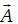(being the magnetic vector potential) satisfies the Ampere-Maxwell equation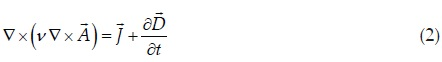where, v is the reluctivity,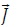is the current density; and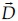= ε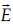being the permittivity) is the electric density. The second term in the Eq. (2) represents the displacement current, which can be dropped if the frequency of the power source is small; in this case, the Eq. (2) is given by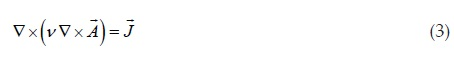The current densityin this equation depends of the type of region (cupper coil, aluminum ring, air or ferromagnetic core) and is given by:

1) Air and ferromagnetic core region

The current density in air and ferromagnetic core regions is=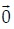; therefore2) Aluminum ring region

The current density= σr(being σr the electric conductivity of the aluminum ring) is found using the Faraday law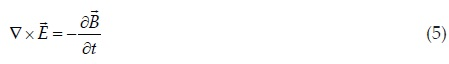Solving this equation for the vector potentialIt is observed that this equation does not contain the term of the scalar electric potential gradient (∇V) due to that there is not a power source in the aluminum region. Substituting Eq. (6) in= σrSubstituting Eq. (6) in Eq. (3)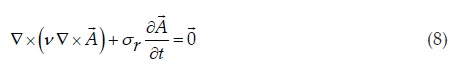3) Copper coil region

The region of the copper coil is modeled as a region that contains N turns where each turn carries the same current ic. In this case, the current density / is uniform with valuewhere, Sc is the cross section area of the copper coil region. Substituting Eq. (9) in Eq. (3)where,is a unit vector pointed in direction of the current density.

Electrical network equations

If the current is known, the solution of the Eq. (10) can be realized. However, this current cannot be known a priori. We know a priori the voltage V between the terminals of the coil. An additional equation is required. This equation is obtained using the Kirchhoff voltage lawwhere, R is the resistance, Φ is the magnetic flux that cross all the turns of the coil. The resistance is given by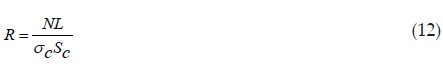where, σc is the electric conductivity of the coil and L is the length of all the turns of the coil. The magnetic flux is given bywhere, the surface Sc comprises all the surfaces of the turns of the coil. Using the fact= ∇ xand the Stokes theorem in Eq. (13) we obtain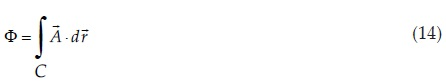where, the trajectory C comprises all the turns of the coil. Substituting Eq. (14) in Eq. (11)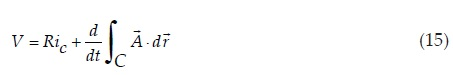Phasor equations

The current in the copper coil is cosine to ensure that the aluminum ring stays in a stationary levitated position. In this situation, the state of the electromagnetic field is stable and the equations of the electromagnetic field can be given in phasor form. In phasor notation, the operator d/dt becomes iw in Eqs. (4), (8) and (10):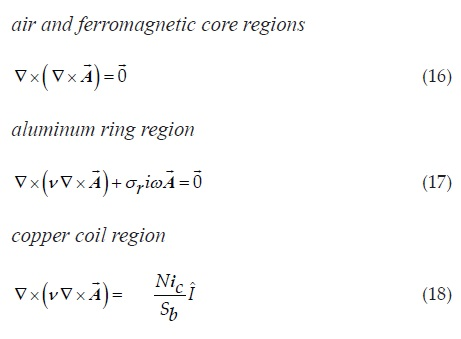where,and ic are phasors of the potentialand the current ic, respectively.

The phasor equation of the electrical network equation Eq. (15) iswhere, V is the phasor of the voltage V.

Boundary conditions

It is observed that Eq. (3) is a second order partial differential equation for the magnetic vector potential. The solution of this partial differential equation requires boundary conditions for the vector potential. The boundary of the solution domain is chosen so that the vector potential can be dropped (magnetic insulation). The magnetic insulation condition is expressed as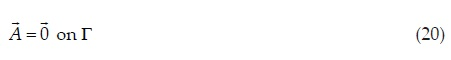where, Γ is the boundary of solution domain. In phasor notation, the condition of magnetic insulation isDiscretization

Using the Galerkin method (Hoole, 1989; Lombard and Meunier, 1992, 1993), Eqs. (16)-(19) can be discretized:where N represents the number of nodes. The matrices and vectors are defined as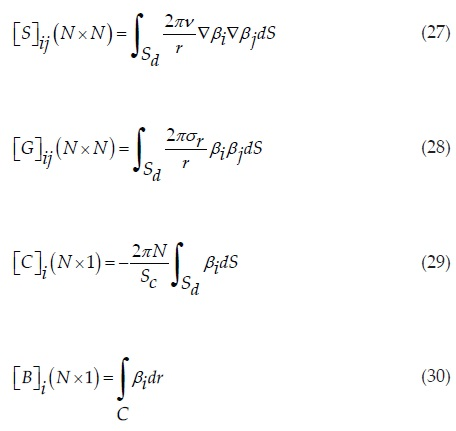where, the vector potential A is expanded in the base function βi: A = ∑βjAj. The surface Sd is the surface of the solution domain.

Mechanical equilibrium

The voltage is a cosine in order to maintain the aluminum ring in a stationary levitated position. This stationary levitation is obtained when the mechanical equilibrium is reached; this is, the Lorente force averaged in a cycle, fzav equals the gravity force fg. Using the complex notation, the Lorente force fzav (Barry and Casey, 1999; Hayt and Buck, 2006) is given bywhere,andare the phasors of magnetic densityand current density, respectively. The factor 1/2 in Eq. (31) is due to that the Lorente force period is half of the magnetic field period (Barry and Casey, 1999). Figure 3 shows the flowchart of the obtaining of the average Lorente force. The steps of this methodology are:1) Calculate the phasor potential using the phasor equations (Eqs. 22-25) along with boundary condition of magnetic insulation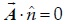on Γ (see Eq. 20).

2) Determine the phasor magnetic density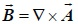and phasor current density(see Eq. 6) in the aluminum ring region.

3) Calculate the average Lorente force(see Eq. 31).

The space distribution of the electromagnetic field depends of the separation sz between the aluminum ring and the copper coil. Therefore, the average Lorente force fzav is a function of the separation (fz = fz(sz)). In order to reach the stationary levitation of the aluminum ring, the average Lorente force fzav equals to the gravity force fg.where, zs' is the separation in stationary levitation and represents the root of Eq. (33). It is observed that Eq. (33) is a transcendental equation. The root of this transcendental equation can be found using a variant of the Newton-Raphson method: secant method (Arfken and Weber, 2005). The convergence of Newton-Raphson is guaranteed due to that the average Lorentz force fzaw(zs) is a function decreasing of the separation zs (see Figure 5). The secant method is defined by the recurrence relation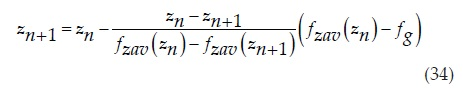Experimental validation

In this section we compared the numerical and experimental results for the separation in stationary levitation zs' as a function of the voltage amplitude in rms,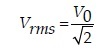. The experimental setup was described in the second section. The numerical results are obtained using the proposed methodology in the section above. Figure 4 shows the separation zs' as function of the voltage amplitude Vrms for both experimental and numerical results. The discrepancy between the theoretical and experimental data is at most 12%. This difference can be due to the fact that the numerical modeling does not take into account the temperature effect in the electric conductivity σ.

Results and discussion

In this section some results obtained by the proposed modeling are studied. The average Lorente force is examined as a function of the separation distance; the ratio between coil current and ring current, and the spatial distribution of the magnetic field.

Figure 5 depicts the average Lorente force fzav as function of the separation zs for a representative voltage amplitude Vrms = 120 V. It is observed that the Lorente force is a decreasing function of the distance zs. This guarantees the convergence of the Newton-Raphson method due to that the derivative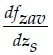is negative.

Figure 6 shows the spatial distribution of the radial component Br0 of the magnetic density amplitude, for a representative voltage amplitude Vrms = 120 V in state of stationary levitation (zs' = 0.057 m). It also presents the positions of the ferromagnetic core, copper coil and aluminum ring. This Figure 6 shows that the radial component is higher in regions close to the core, coil and ring edges. In contrast, the radial component Br0 presents small values in positions far away from above edges.The total current in the ring ir is realized by means of;while the total current in the region of the coil is Nic. In Figure 7 is shown the ratioas function of voltage amplitude Vrms in stationary levitation. It is observed that the highest value (ir / Nic = 0.47) occurs in Vrms = 43.4 V corresponding to a separation zs' = 0. The ratio ir / Nic decreases if the voltage amplitude Vrms increases. Also, in a first order approach, the magnetic field originated by any system is proportional to the current of this system. Therefore, the magnetic coupling of the ring on the coil can be neglected for high values of voltage amplitude.Conclusions

The aim of this work was to present a numerical modeling based upon the use of the Galerkin method to simulate the electromagnetic field of the Thomson ring. Also, this modeling is capable of simulating numerically the separation between aluminum ring and copper coil in situation of stationary levitation (the average Lorente force equals gravity force). This calculation of the separation uses the Newton-Raphson method.

The proposed modeling was validated comparing theoretical and experimental results. The compared results were the separation between the aluminum ring and the copper coil (in stationary levitation) for different voltage amplitudes.

It is concluded that the magnetic coupling of the aluminum ring on the coil can be neglected if the source voltage is high. Therefore, the coil current can be modeled without taking into account the coupling ring-coil. This means that the coil current is found using a RL (resistance-inductance) circuit; where, the resistance and inductance are parameter of the coil.

References

Alferov D,. Budovsky A., Evsin D., Ivanov V., Sidorov V., Yagnov V. DC vacuum circuit-breaker, in: International Symposium on Discharges and Electrical Insulation in Vacuum, 24th, Bucharest, 2008, pp. 173-176.         [ Links ]

Arfken G.B., Weber H.J. Mathematical methods for physicists, sixth ed., New York, Elsevier, Academic Press, 2005.         [ Links ]

Barry N., Casey R. Elihu Thomson's jumping ring in a levitated closed-loop control experiment, Education. IEEE Transactions on, volume 42 (issue 1), 1999: 72-80.         [ Links ]

Belforte P., Chiampi M., Tartaglia M. A finite element computation procedure for electromagnetic fields under different supply conditions, Magnetics. IEEE Transactions on, volume 21 (issue 6), 1985: 2284-2287.         [ Links ]

Bissal A., Salinas E., Engdahl G., Ohrstrom M. Simulation and verification of Thomson actuator systems, in Proceedings of the COMSOL Conference, Paris, France, 2010.         [ Links ]

COMSOL, AC/DC MODULE User's Guide, 2008.         [ Links ]

Hayt W.H., Buck J.A. Teoría electromagnética, New York, Me Graw Hill, 2006.         [ Links ]

Hoole S.E.H. Computer aided analysis and design of electromagnetic devices, New York, Elsevier, 1989.         [ Links ]

Konrad A. Integrodifferential finite element formulation of two-dimensional steady-state skin effect problems. IEEE Transactions on Magnetics, volume 18 (issue 1), 1982: 284-292.         [ Links ]

Li W., Jeong Y.W., Yoon H.S., Koh C.S. Analysis of parameters influence on the characteristics of Thomson coil type actuator of arc eliminator using adaptive segmentation equivalent circuit method. Journal of Electrical Engineering & Technology, volume 5 (issue 2), 2010: 282-289.         [ Links ]

Lombard P., Meunier G. A general method for electric and magnetic coupled problem in 2D and magnetodynamic domain. IEEE Transactions on Magnetics, volume 28 (issue 2), 1992: 1291-1294.         [ Links ]

Lombard P., Meunier G. A general purpose method for electric and magnetic combined problems for 2D, axisymmetric and transient systems. IEEE Transactions on Magnetics, volume 29 (issue 2), 1993: 1737-1740.         [ Links ]

Meunier G, Shen D., Coulomb J.L. Modelisation of 2D and axisymmetric magnetodynamic domain by the finite elements method. IEEE Transactions on Magnetics, volume 24 (issue 1), 1998: 166-169.         [ Links ]

Meyer J.M., Rufer A. A DC hybrid circuit breaker with ultra-fast contact opening and integrated gate-commutated thyristors (IGCTs). IEEE Transactions on Power Delivery, volume 21 (issue 2), 2006: 646-651.         [ Links ]

Patitsas S.N. Stability analysis for axially-symmetric magnetic field levitation of a superconducting sphere. Physica C, volume 471 (issues 1-2), 2011: 12-18.         [ Links ]

Piriou F., Razek A. Simulation of electromagnetic systems by coupling of magnetic and electric equations. Mathematics and Computers in Simulation, volume 31 (issue 3), 1989: 189-194.         [ Links ]

Citation for this article:

Chicago citation style
Guzmán, Juan, Felipe de Jesús González-Montañez, Rafael Escarela-Pérez, Juan Carlos Olivares-Galván, Victor Manuel Jiménez-Mondragon. Numerical modeling of the Thomson ring in stationary levitation using FEM-electrical network and Newton-Raphson. Ingeniería Investigación y Tecnología, XVI, 03 (2015): 431-439.

ISO 690 citation style
Guzmán J., González-Montañez F.J., Escarela-Pérez R., Olivares-Galván J.C., Jiménez-Mondragon V.M. Numerical modeling of the Thomson ring in stationary levitation using FEM-electrical network and Newton-Raphson. Ingeniería Investigación y Tecnología, volume XVI (issue 3), july 2015: 431-439.

About the authors

Juan Guzman. Obtained Ph.D. in Energy Engineering from the Universidad Nacional Autónoma de México, México City, Mexico, in 2008. He is currently with the área de ingeniería energética y electromagnética, Departamento de Energía, UAM, Azcapotzalco, México.

Felipe de Jesús González-Montañez. He received the M.Sc. degree in electrical engineering from the Centro de Investigación y de Estudios Avanzados del IPN, México City, Mexico, in 2011. His research interests include the modeling and control of electrical machines.

Rafael Escarela-Pérez. He obtained his B.Sc. in electrical engineering from Universidad Autónoma Metropolitana, Mexico City in 1992 and his Ph.D. from Imperial College, London in 1996. He is interested in the modeling of electrical machines.

Juan Carlos Olivares-Galván. He received the Ph.D. degree in electrical engineering from CINVESTAV, Guadalajara, Mexico, in 2003. His main research interests are related to the experimental and numerical analysis of electromagnetic devices.

Victor Manuel Jiménez-Mondragon. He received the M.Sc. degree in electrical engineering from the Universidad Nacional Autónoma de México, México City, Mexico, in 2012. He is interested in the modeling of electrical machines.All the contents of this journal, except where otherwise noted, is licensed under a Creative Commons Attribution License